##1. Triangles inscribed in rectangular hyperbolas

A triangle having its vertices A, B, C on a rectangular hyperbola is said inscribed in it. Such a triangle has:
1) Its Euler circle passing through the center O of the hyperbola.
2) Its Orthocenter H lying also on the hyperbola.
3) The fourth intersection point N of the circumcircle with the hyperbola symmetric to H w.r. to O.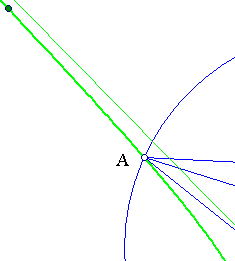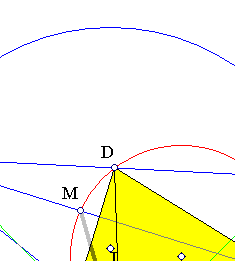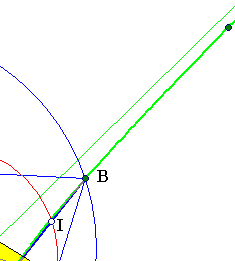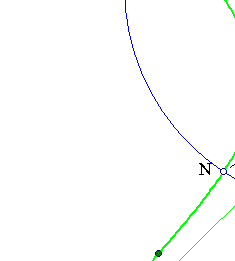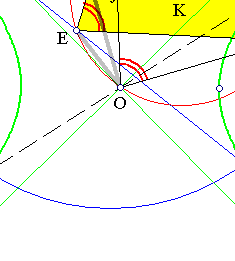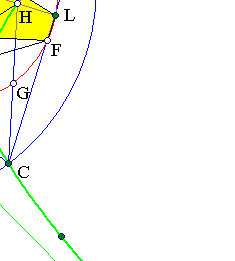The clue fact is that the angle of two lines is equal to the angle of their conjugates (discussed in RectangularAsProp.html ). This implies that the angle of two chords is equal to the angle of the lines joining their middles with the center O of the hyperbola. Consider now triangle ABC inscribed in the rectangular hyperbola and draw its height AL. Define H to be the intersection point of AL with the hyperbola. We want to show that H is the orthocenter. Join H to the other vertices and define the middles G, I, E, D, M, F of the segments CH, CB, HB, ...etc.
It is well known that the quadrilateral DEFL is cyclic and its circumcircle is the Euler circle. By the clue-fact refered above, angle(DOF) = angle(DEF), hence O belongs to the Euler circle. This proves (1).
Consider now the middle M of AH and the intersection point M' of AH with the Euler circle. For M' we know that angle(EOM') = angle(ELM') = angle(EAM'). For M, by the clue-fact, we know that also angle(EOM) = angle(EAM') = angle(EAM). Thus M and M' make equal angles to OE, hence they coincide. The Euler circle passes through M and H is the orthocenter. This proves (2).
The third assertion is a consequence of the first two, since the Euler circle is homothetic w.r to the orthocenter by a factor of (1/2) and the hyperbola is symmetric w.r. to its center O.

Remark The discussion leads to the way to construct all rectangular hyperbolas circumscribing the triangle ABC. Simply select a point O on the Euler circle to serve as the center of the hyperbola. The hyperbola can be constructed as a conic passing through five points {A,B,C,H,A'}, A' being the symmetric of A with respect to O.

##2. Generating equilaterals

Take a point H on an equilateral hyperbola and draw a circle centered at H and passing through the symmetric H' of H with respect to the center O of the hyperbola. The other three intersection points {A,B,C} of the circle with the hyperbola make an equilateral triangle.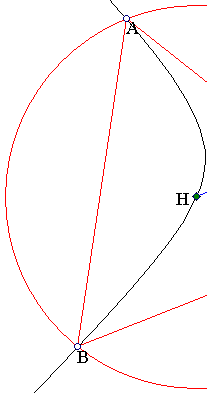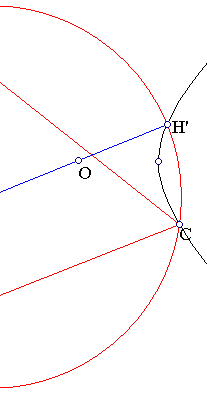By (3) of the first proposition, H is the orthocenter of the triangle. Since it is also the circumcenter, the triangle is equilateral.

AntiparallelHyperbola.html
AsymptoticTriangle.html
Hyperbola.html
HyperbolaAsymptoticProperty.html
HyperbolaAsymptotics.html
HyperbolaRectangular.html
Orthic.html
RectangularAsProp.html
RectHypeParaChords.html
RectHyperbola.html
RectHypeRelation.html

### References

Deltheil, R & Caire, D. Geometrie et Complements Paris, Editions Gabay, 1989, p. 259.